#### Howdy, Stranger!

It looks like you're new here. If you want to get involved, click one of these buttons!

Options

# Lecture 71 - Chapter 4: Caps and Cups for Enriched Profunctors

edited February 17

Last time we saw how to tensor enriched profunctors. Now let's see caps and cups for enriched profunctors! We are building up to a kind of climax of this course: the theory of 'compact closed categories'. This is the right language for studying many kinds of complex systems, from collaborative design and PERT charts to electrical circuits and control theory. But we need to climb the mountain patiently.

We've already seen caps and cups for feasibility relations in Lecture 68. We can just generalize what we did.

As usual, let's assume $$\mathcal{V}$$ is a commutative quantale, so we get a category $$\mathbf{Prof}_\mathcal{V}$$ where:

• objects are $$\mathcal{V}$$-enriched categories

and

• morphisms are $$\mathcal{V}$$-enriched profunctors.

To keep my hands from getting tired, from now on in this lecture I'll simply write 'enriched' when I mean '$$\mathcal{V}$$-enriched'.

Let $$\mathcal{X}$$ be an enriched category. Then there's an enriched profunctor called the cup

$$\cup_{\mathcal{X}} \colon \mathcal{X}^{\text{op}} \otimes \mathcal{X} \nrightarrow \textbf{1}$$ drawn as follows: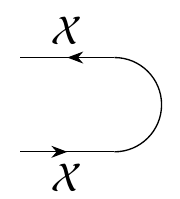To define it, remember that enriched profunctors $$\mathcal{X}^{\text{op}} \otimes \mathcal{X} \nrightarrow \textbf{1}$$ are really just enriched functors $$(\mathcal{X}^{\text{op}} \otimes \mathcal{X})^\text{op} \otimes \textbf{1} \to \mathcal{V}$$. Also, remember that $$\mathcal{X}$$ comes with a hom-functor, which is the enriched functor

$$\mathrm{hom} \colon \mathcal{X}^{\text{op}} \otimes \mathcal{X} \to \mathcal{V}$$ sending any object $$(x,x')$$ to $$\mathcal{X}(x,x')$$. So, we define $$\cup_\mathcal{X}$$ to be the composite

$$(\mathcal{X}^{\text{op}} \otimes \mathcal{X})^\text{op} \otimes \textbf{1} \stackrel{\sim}{\to} (\mathcal{X}^{\text{op}} \otimes \mathcal{X})^\text{op} \stackrel{\sim}{\to} (\mathcal{X}^{\text{op}})^\text{op} \otimes \mathcal{X}^{\text{op}} \stackrel{\sim}{\to} \mathcal{X} \otimes \mathcal{X}^{\text{op}} \stackrel{\sim}{\to} \mathcal{X}^{\text{op}} \otimes \mathcal{X} \stackrel{\text{hom}}{\to} \mathcal{V}$$ where the arrows with squiggles over them are isomorphisms, most of which I explained last time.

There's also an enriched profunctor called the cap

$$\cap_\mathcal{X} \colon \textbf{1} \nrightarrow \mathcal{X} \otimes \mathcal{X}^{\text{op}}$$ drawn like this: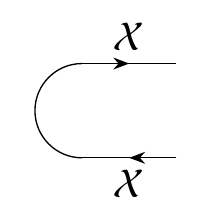To define this, remember that enriched profunctors $$\textbf{1} \nrightarrow \mathcal{X} \otimes \mathcal{X}^{\text{op}}$$ are enriched profunctors $$\textbf{1}^{\text{op}} \otimes (\mathcal{X} \otimes \mathcal{X}^{\text{op}})$$. But $$\textbf{1}^{\text{op}} = \textbf{1}$$, so we define the cap to be the composite

$$\textbf{1}^{\text{op}} \otimes (\mathcal{X} \otimes \mathcal{X}^{\text{op}}) = \textbf{1}\otimes (\mathcal{X} \otimes \mathcal{X}^{\text{op}}) \stackrel{\sim}{\to} \mathcal{X} \otimes \mathcal{X}^{\text{op}} \stackrel{\sim}{\to} \mathcal{X}^{\text{op}} \otimes \mathcal{X} \stackrel{\text{hom}}{\to} \mathcal{V} .$$ As we've already seen for feasibility relations, the cap and cup obey the snake equations, also known as zig-zag equations or yanking equations. (Everyone likes making up their own poetic names for these equations.) The first snake equation says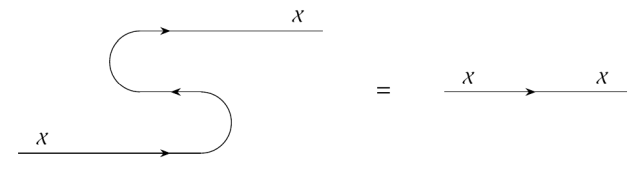In other words, the composite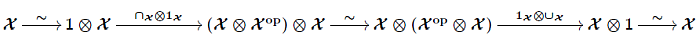is the identity, where the arrows with squiggles over them are obvious isomorphisms that I described last time. The second snake equation says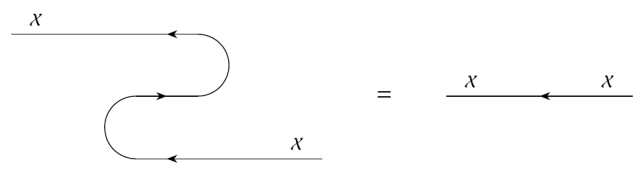In other words, the composite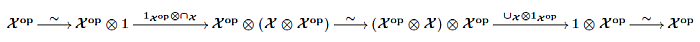is the identity.

Last time I sketched how $$\mathbf{Prof}_{\mathcal{V}}$$ is a monoidal category, meaning one with a tensor product obeying certain rules. It's also symmetric monoidal, meaning it has isomorphisms

$$\sigma_{\mathcal{X}, \mathcal{Y}} \colon \mathcal{X} \otimes \mathcal{Y} \nrightarrow \mathcal{Y} \otimes \mathcal{X}$$ obeying certain rules. These let us switch the order of objects in a tensor product: in terms of diagrams, it means wires can cross each other! And finally, when every object in a symmetric monoidal category has a cap and cup obeying the snake equations, we say that category is compact closed. I will define all these concepts more carefully soon. For now I just want you to know that

Compact closed categories let us study processes that can run in series or in parallel, with feedback.

and also that $$\mathbf{Prof}_{\mathcal{V}}$$ is an example of a compact closed category. If you're impatient to learn more, try Section 4.4 of the book.

Puzzle 227. Prove the snake equations in $$\mathbf{Prof}_{\mathcal{V}}$$.

For this, I should state the snake equations more precisely! The first one says this composite: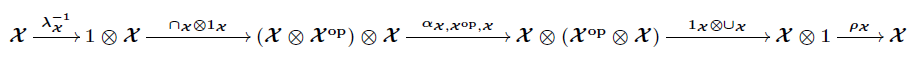is the identity, where $$\alpha$$ is the associator and $$\lambda, \rho$$ are the left and right unitors, defined last time. The second snake equation says this composite: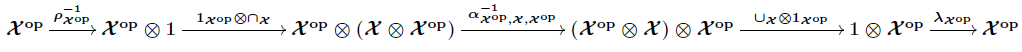is the identity.

To read other lectures go here.

• Options
1.
edited August 2018

Let $$x\in\mathcal{X}$$.

$$\lambda_\mathcal{X}^{-1}(x)=I\otimes x.$$ $$( \cap_\mathcal{X}\otimes1_\mathcal{X})(I\otimes x)=\bigvee_{y\in\mathcal{X}}(y\otimes y^\mathrm{op})\otimes x .$$ $$\alpha_\mathcal{X,X^\mathrm{op},X}(\bigvee_{y\in\mathcal{X}}(y\otimes y^\mathrm{op})\otimes x)=\bigvee_{y\in\mathcal{X}}y\otimes(y^\mathrm{op}\otimes x).$$ $$(1_\mathcal{X}\otimes\cup_\mathcal{X})(\bigvee_{y\in\mathcal{X}}y\otimes(y^\mathrm{op}\otimes x))=(x\otimes I)\vee(\bigvee_{y\neq x}y\otimes0)=(x\otimes I)\vee 0=x\otimes I.$$ $$\rho_\mathcal{X}(x\otimes I)=x.$$ So, the first composite is the identity.

Let $$x\in\mathcal{X^\mathrm{op}}$$.

$$\rho_\mathcal{X^\mathrm{op}}^{-1}(x)=x\otimes I.$$ $$(1_\mathcal{X}\otimes\cap_\mathcal{X})(x\otimes I)=\bigvee_{y\in\mathcal{X}}x\otimes(y\otimes y^\mathrm{op}).$$ $$\alpha_\mathcal{X^\mathrm{op},X,X^\mathrm{op}}^{-1}(\bigvee_{y\in\mathcal{X}}x\otimes(y\otimes y^\mathrm{op}))=\bigvee_{y\in\mathcal{X}}(x\otimes y)\otimes y^\mathrm{op}.$$ $$(\cup_\mathcal{X}\otimes1_\mathcal{X^\mathrm{op}})(\bigvee_{y\in\mathcal{X}}(x\otimes y)\otimes y^\mathrm{op})=(I\otimes x)\vee(\bigvee_{y\neq x}0\otimes y^\mathrm{op})=(I\otimes x)\vee 0=I\otimes x .$$ $$\lambda_\mathcal{X^\mathrm{op}}(I\otimes x)=x.$$ So, the second composite is the identity as well.

Comment Source:Let \$$x\in\mathcal{X}\$$. $\lambda_\mathcal{X}^{-1}(x)=I\otimes x.$ $( \cap\_\mathcal{X}\otimes1\_\mathcal{X})(I\otimes x)=\bigvee\_{y\in\mathcal{X}}(y\otimes y^\mathrm{op})\otimes x .$ $\alpha_\mathcal{X,X^\mathrm{op},X}(\bigvee\_{y\in\mathcal{X}}(y\otimes y^\mathrm{op})\otimes x)=\bigvee\_{y\in\mathcal{X}}y\otimes(y^\mathrm{op}\otimes x).$ $(1\_\mathcal{X}\otimes\cup\_\mathcal{X})(\bigvee\_{y\in\mathcal{X}}y\otimes(y^\mathrm{op}\otimes x))=(x\otimes I)\vee(\bigvee\_{y\neq x}y\otimes0)=(x\otimes I)\vee 0=x\otimes I.$ $\rho_\mathcal{X}(x\otimes I)=x.$ So, the first composite is the identity. Let \$$x\in\mathcal{X^\mathrm{op}}\$$. $\rho_\mathcal{X^\mathrm{op}}^{-1}(x)=x\otimes I.$ $(1\_\mathcal{X}\otimes\cap\_\mathcal{X})(x\otimes I)=\bigvee\_{y\in\mathcal{X}}x\otimes(y\otimes y^\mathrm{op}).$ $\alpha_\mathcal{X^\mathrm{op},X,X^\mathrm{op}}^{-1}(\bigvee\_{y\in\mathcal{X}}x\otimes(y\otimes y^\mathrm{op}))=\bigvee\_{y\in\mathcal{X}}(x\otimes y)\otimes y^\mathrm{op}.$ $(\cup\_\mathcal{X}\otimes1\_\mathcal{X^\mathrm{op}})(\bigvee\_{y\in\mathcal{X}}(x\otimes y)\otimes y^\mathrm{op})=(I\otimes x)\vee(\bigvee\_{y\neq x}0\otimes y^\mathrm{op})=(I\otimes x)\vee 0=I\otimes x .$ $\lambda_\mathcal{X^\mathrm{op}}(I\otimes x)=x.$ So, the second composite is the identity as well.
• Options
2.
edited August 2018

Hi, David! Most of your calculations look good to me; I added a bit of extra space to make them easier for me to read.

But you seem to be using some formulas for $$\cap_X$$ and $$\cup_X$$ that I don't know. These guys are not enriched functors; they're enriched profunctors, so you can't simply apply them to an object and get another object. For example $$\cap_X$$ is an enriched profunctor

$$\cap_X \colon \textbf{1} \nrightarrow \mathcal{X} \otimes \mathcal{X}^{\text{op}}$$ but you seem to be applying it to the object $$1 \in \mathbf{1}$$ and getting

$$\bigvee_{y \in \mathcal{X}} y^{\text{op}} \otimes y$$ I don't know what this expression even means! $$y$$ is an object in $$\mathcal{X}$$, $$y^{\text{op}}$$ is a perfectly fine name for the corresponding object in $$\mathcal{X}^{\text{op}}$$ (which, remember, has the same set of objects. I don't know what it means to tensor an object of $$\mathcal{X}$$ and one of $$\mathcal{X}^{\text{op}}$$, but I can make something up: the objects of $$\mathcal{X} \otimes \mathcal{X}^{\text{op}}$$ are pairs $$(x,y)$$ consisting of an object in $$\mathcal{X}$$ and one in $$\mathcal{X}^{\text{op}}$$; if we were in a certain mood we could write such a pair as $$x \otimes y$$. The worst problem comes at the end: I don't what it means to take the join of a bunch of such tensor products. I can take joins in a poset, like $$\mathcal{V}$$, but $$\mathcal{X} \otimes \mathcal{X}^{\text{op}}$$ is not a poset.

It's possible you're doing something correct, but I'd need some more explanation to understand what

$$\bigvee_{y \in \mathcal{X}} y^{\text{op}} \otimes y$$ means and why we should think of $$\cap_X$$ as sending $$1 \in \mathbf{1}$$ to this thing.

Comment Source:Hi, David! Most of your calculations look good to me; I added a bit of extra space to make them easier for me to read. But you seem to be using some formulas for \$$\cap_X\$$ and \$$\cup_X\$$ that I don't know. These guys are not enriched functors; they're enriched profunctors, so you can't simply apply them to an object and get another object. For example \$$\cap_X\$$ is an enriched profunctor $\cap_X \colon \textbf{1} \nrightarrow \mathcal{X} \otimes \mathcal{X}^{\text{op}}$ but you seem to be applying it to the object \$$1 \in \mathbf{1}\$$ and getting $\bigvee_{y \in \mathcal{X}} y^{\text{op}} \otimes y$ I don't know what this expression even means! \$$y\$$ is an object in \$$\mathcal{X}\$$, \$$y^{\text{op}}\$$ is a perfectly fine name for the corresponding object in \$$\mathcal{X}^{\text{op}}\$$ (which, remember, has the same set of objects. I don't know what it means to tensor an object of \$$\mathcal{X}\$$ and one of \$$\mathcal{X}^{\text{op}}\$$, but I can make something up: the objects of \$$\mathcal{X} \otimes \mathcal{X}^{\text{op}}\$$ are pairs \$$(x,y)\$$ consisting of an object in \$$\mathcal{X}\$$ and one in \$$\mathcal{X}^{\text{op}}\$$; if we were in a certain mood we could write such a pair as \$$x \otimes y\$$. The worst problem comes at the end: I don't what it means to take the join of a bunch of such tensor products. I can take joins in a poset, like \$$\mathcal{V}\$$, but \$$\mathcal{X} \otimes \mathcal{X}^{\text{op}}\$$ is not a poset. It's possible you're doing something correct, but I'd need some more explanation to understand what $\bigvee_{y \in \mathcal{X}} y^{\text{op}} \otimes y$ means and why we should think of \$$\cap_X\$$ as sending \$$1 \in \mathbf{1}\$$ to this thing.
• Options
3.
edited August 2018

Ah, ok, I see now that I should have used the function on objects induced by the profunctor $$\cap_\mathcal{X}$$. So, the first diagram chase should say:

Let $$x\in\mathcal{X}$$.

$$\lambda_\mathcal{X}^{-1}(x)=1\otimes x.$$ $$( \cap_\mathcal{X}\otimes1_\mathcal{X})(1\otimes x)=(x\otimes x)\otimes x .$$ $$\alpha_\mathcal{X,X^\mathrm{op},X}((x\otimes x)\otimes x)=x\otimes(x\otimes x).$$ $$(1_\mathcal{X}\otimes\cup_\mathcal{X})(x\otimes(x\otimes x))=x\otimes1.$$ $$\rho_\mathcal{X}(x\otimes\mathrm{id}_{x})=x.$$ , and the second one should be similarly modified.

I guess I was confused by the fact that I expected $$\cap_\mathcal{X}(x)$$ to give the same result indepedent of $$x\in\mathcal{X}$$, because I had in mind insertion of the identity operator in quantum mechanics.

Comment Source:Ah, ok, I see now that I should have used the function on objects induced by the profunctor \$$\cap_\mathcal{X}\$$. So, the first diagram chase should say: Let \$$x\in\mathcal{X}\$$. $\lambda_\mathcal{X}^{-1}(x)=1\otimes x.$ $( \cap\_\mathcal{X}\otimes1\_\mathcal{X})(1\otimes x)=(x\otimes x)\otimes x .$ $\alpha_\mathcal{X,X^\mathrm{op},X}((x\otimes x)\otimes x)=x\otimes(x\otimes x).$ $(1\_\mathcal{X}\otimes\cup\_\mathcal{X})(x\otimes(x\otimes x))=x\otimes1.$ $\rho_\mathcal{X}(x\otimes\mathrm{id}_{x})=x.$ , and the second one should be similarly modified. I guess I was confused by the fact that I expected \$$\cap_\mathcal{X}(x)\$$ to give the same result indepedent of \$$x\in\mathcal{X}\$$, because I had in mind insertion of the identity operator in quantum mechanics.
• Options
4.
edited August 2018

Sorry, David: a profunctor typically does not give rise to a function on objects. In particular,

$$\cap_{\mathcal{X}} (1) = x \otimes x$$ does not make sense. And there's another way to see something is wrong here: what's $$x$$ supposed to be on the right-hand side? There's no $$x$$ on the left-hand side!

A profunctor that's the companion of a functor does gives rise to a function on objects. An example would be the associator. But the cap and the cup are not companions of anything.

I think I've failed to adequately explain how to compose profunctors. I gave the formula in Lecture 63, and people solved some puzzles based on this, but when it comes to more complicated applications, like Puzzle 227 in this lecture, people seem to be stuck.

I'll start by reminding everyone of the formula:

Definition. If $$\mathcal{V}$$ is a commutative quantale and $$\Phi \colon \mathcal{X} \nrightarrow \mathcal{Y}$$, $$\Psi\colon \mathcal{Y} \nrightarrow \mathcal{Z}$$ are $$\mathcal{V}$$-enriched profunctors, define their composite by

$$(\Psi\Phi)(x,z) = \bigvee_{y \in \mathrm{Ob}(\mathcal{Y})} \Phi(x,y) \otimes \Psi(y,z).$$

So, profunctors are like matrices, and the formula for composing them is like matrix multiplication, with $$\otimes$$ replacing multiplication, and $$\bigvee$$ replacing $$\sum$$. Remember, matrix multiplication goes like this:

$$(AB)_{i k} = \sum_j A_{i j} B_{j k} .$$ It's the same basic idea.

Comment Source:Sorry, David: a profunctor typically does not give rise to a function on objects. In particular, $\cap_{\mathcal{X}} (1) = x \otimes x$ does not make sense. And there's another way to see something is wrong here: what's \$$x\$$ supposed to be on the right-hand side? There's no \$$x\$$ on the left-hand side! A profunctor that's the companion of a functor _does_ gives rise to a function on objects. An example would be the associator. But the cap and the cup are not companions of anything. I think I've failed to adequately explain how to compose profunctors. I gave the formula in [Lecture 63](https://forum.azimuthproject.org/discussion/2295/lecture-63-chapter-4-composing-enriched-profunctors/p1), and people solved some puzzles based on this, but when it comes to more complicated applications, like Puzzle 227 in this lecture, people seem to be stuck. I'll start by reminding everyone of the formula: > **Definition.** If \$$\mathcal{V}\$$ is a commutative quantale and \$$\Phi \colon \mathcal{X} \nrightarrow \mathcal{Y}\$$, \$$\Psi\colon \mathcal{Y} \nrightarrow \mathcal{Z}\$$ are \$$\mathcal{V}\$$-enriched profunctors, define their **composite** by > $(\Psi\Phi)(x,z) = \bigvee_{y \in \mathrm{Ob}(\mathcal{Y})} \Phi(x,y) \otimes \Psi(y,z).$ So, profunctors are like matrices, and the formula for composing them is like matrix multiplication, with \$$\otimes\$$ replacing multiplication, and \$$\bigvee\$$ replacing \$$\sum\$$. Remember, matrix multiplication goes like this: $(AB)\_{i k} = \sum\_j A_\{i j} B\_{j k} .$ It's the same basic idea.
• Options
5.

I will soon move on from this enriched profunctor business and talk about other things, but it'll be good if some of you folks can prove the snake equations to make me feel you've got enriched profunctors down pat.

Comment Source:I will soon move on from this enriched profunctor business and talk about other things, but it'll be good if some of you folks can prove the snake equations to make me feel you've got enriched profunctors down pat.
• Options
6.
edited August 2018

David Lambert, we want Bool-functors. This means that secretly we want monotone functions that have the poset Bool as the codomain.

This means that a Bool-profunctor,

$X \nrightarrow Y$

is secretly a monotone function,

$X \otimes Y \to \mathbf{Bool}.$

So for the inverse left unitor Bool-profunctor (say that 10-times fast),

$$\lambda_\mathcal{X}^{-1} : X \nrightarrow 1\otimes X.$$ we actually have something like,

$\lambda_{X}^{-1}: X\otimes(1\otimes X) \to \mathbf{Bool} \\ \lambda_{X}^{-1}((x),(0,x)) = \begin{cases} \texttt{true} & \text{ if } x \leq (0,x) \\ \texttt{false} & \text{otherwise} \end{cases}$

Comment Source:David Lambert, we want ***Bool***-functors. This means that secretly we want monotone functions that have the poset **Bool** as the codomain. This means that a **Bool**-profunctor, \$X \nrightarrow Y \$ is secretly a monotone function, \$X \otimes Y \to \mathbf{Bool}. \$ So for the inverse left unitor **Bool**-profunctor (say that 10-times fast), $\lambda_\mathcal{X}^{-1} : X \nrightarrow 1\otimes X.$ we actually have something like, \$\lambda_{X}^{-1}: X\otimes(1\otimes X) \to \mathbf{Bool} \\\\ \lambda_{X}^{-1}((x),(0,x)) = \begin{cases} \texttt{true} & \text{ if } x \leq (0,x) \\\\ \texttt{false} & \text{otherwise} \end{cases} \$
• Options
7.
edited August 2018

I think that we have the following definitions for the profunctors involved in the first snake equation:

\begin{align} \lambda_{\mathcal{X}}^{-1}(a, \langle 1, b \rangle) &= \mathcal{X}(a, b) \\ (\cap_\mathcal{X} \otimes 1_\mathcal{X})(\langle 1, b \rangle, \langle \langle c, c' \rangle, c'' \rangle) &= \mathcal{X}(c', c) \otimes \mathcal{X}(b, c'') \\ \alpha_{\mathcal{X}, \mathcal{X}^\mathrm{op}, \mathcal{X}}(\langle \langle c, c' \rangle, c'' \rangle, \langle d, \langle d', d'' \rangle \rangle) &= \mathcal{X}(c, d) \otimes \mathcal{X}(d', c') \otimes \mathcal{X}(c'', d'') \\ (1_\mathcal{X} \otimes \cup_\mathcal{X})(\langle d, \langle d', d'' \rangle \rangle, \langle e, 1 \rangle) &= \mathcal{X}(d, e) \otimes \mathcal{X}(d'', d') \\ \rho_\mathcal{X}(\langle e, 1 \rangle, f) &= \mathcal{X}(e, f). \\ \end{align} Using the definition of composition and the fact that $$\mathcal{V}$$ is a commutative quantale, we get the composition of the above profunctors to be

$$\bigvee_{b, c, c', c'', d, d', d'', e} \mathcal{X}(a, b) \otimes \mathcal{X}(c', c) \otimes \mathcal{X}(b, c'') \otimes \mathcal{X}(c, d) \otimes \mathcal{X}(d', c') \otimes \mathcal{X}(c'', d'') \otimes \mathcal{X}(d, e) \otimes \mathcal{X}(d'', d') \otimes \mathcal{X}(e, f).$$ This equation is equal to the idenity profunctor, $$\mathcal{X}(a, f)$$, if

$$\bigvee_{y} \mathcal{X}(x, y) \otimes \mathcal{X}(y, z) = \mathcal{X}(x, z).$$ But I don't quite know how to show this – have we already proven this equation?

Edit: I think I know how to prove the last equation. We can use the two properties of the join:

• The join $$j$$ is an upper bound; so for any $$y$$ we have that $$\mathcal{X}(x, y) \otimes \mathcal{X}(y, z) \le j$$. If we pick $$y$$ to be $$x$$ then we get that $$\mathcal{X}(x,z) \le j$$.
• The join $$j$$ is the least upper bound; so for any other upper bound $$u$$ we have that $$j \le u$$. Since $$\mathcal{X}(x,y) \otimes \mathcal{X}(y,z) \le \mathcal{X}(x, z)$$ for all $$y$$, then $$\mathcal{X}(x,z)$$ is an upper bound and $$j \le \mathcal{X}(x, z)$$.

Hence, the join is exactly $$\mathcal{X}(x,z)$$.

Comment Source:I think that we have the following definitions for the profunctors involved in the first snake equation: \begin{align} \lambda_{\mathcal{X}}^{-1}(a, \langle 1, b \rangle) &= \mathcal{X}(a, b) \\\\ (\cap\_\mathcal{X} \otimes 1\_\mathcal{X})(\langle 1, b \rangle, \langle \langle c, c' \rangle, c'' \rangle) &= \mathcal{X}(c', c) \otimes \mathcal{X}(b, c'') \\\\ \alpha_{\mathcal{X}, \mathcal{X}^\mathrm{op}, \mathcal{X}}(\langle \langle c, c' \rangle, c'' \rangle, \langle d, \langle d', d'' \rangle \rangle) &= \mathcal{X}(c, d) \otimes \mathcal{X}(d', c') \otimes \mathcal{X}(c'', d'') \\\\ (1\_\mathcal{X} \otimes \cup\_\mathcal{X})(\langle d, \langle d', d'' \rangle \rangle, \langle e, 1 \rangle) &= \mathcal{X}(d, e) \otimes \mathcal{X}(d'', d') \\\\ \rho_\mathcal{X}(\langle e, 1 \rangle, f) &= \mathcal{X}(e, f). \\\\ \end{align} Using the definition of composition and the fact that \$$\mathcal{V}\$$ is a commutative quantale, we get the composition of the above profunctors to be $\bigvee_{b, c, c', c'', d, d', d'', e} \mathcal{X}(a, b) \otimes \mathcal{X}(c', c) \otimes \mathcal{X}(b, c'') \otimes \mathcal{X}(c, d) \otimes \mathcal{X}(d', c') \otimes \mathcal{X}(c'', d'') \otimes \mathcal{X}(d, e) \otimes \mathcal{X}(d'', d') \otimes \mathcal{X}(e, f).$ This equation is equal to the idenity profunctor, \$$\mathcal{X}(a, f)\$$, if $\bigvee_{y} \mathcal{X}(x, y) \otimes \mathcal{X}(y, z) = \mathcal{X}(x, z).$ But I don't quite know how to show this – have we already proven this equation? *Edit:* I think I know how to prove the last equation. We can use the two properties of the join: - The join \$$j\$$ is an upper bound; so for any \$$y\$$ we have that \$$\mathcal{X}(x, y) \otimes \mathcal{X}(y, z) \le j\$$. If we pick \$$y\$$ to be \$$x\$$ then we get that \$$\mathcal{X}(x,z) \le j\$$. - The join \$$j\$$ is the _least_ upper bound; so for any other upper bound \$$u\$$ we have that \$$j \le u\$$. Since \$$\mathcal{X}(x,y) \otimes \mathcal{X}(y,z) \le \mathcal{X}(x, z)\$$ for all \$$y\$$, then \$$\mathcal{X}(x,z)\$$ is an upper bound and \$$j \le \mathcal{X}(x, z)\$$. Hence, the join is exactly \$$\mathcal{X}(x,z)\$$.
• Options
8.
edited August 2018

Hey, Dan, that stuff looks really good! I'm about to step out so for now just a quick remark. The identity profunctor

$$1_{\mathcal{X}} \colon \mathcal{X} \nrightarrow \mathcal{X}$$ is defined by

$$1_{\mathcal{X}}(x,y) = \mathcal{X}(x,y) .$$ In other words, it's just the hom-functor in disguise. So, this equation:

$$\bigvee_{y} \mathcal{X}(x, y) \otimes \mathcal{X}(y, z) = \mathcal{X}(x, z).$$ says that the identity profunctor composed with itself is the identity profunctor. That's gotta be true!

And indeed, in Lecture 64 there's a proof that the identity profunctor works as advertised: composing it with any other profunctor doesn't do anything. So, that proof gives this equation:

$$\bigvee_{y} \mathcal{X}(x, y) \otimes \mathcal{X}(y, z) = \mathcal{X}(x, z).$$ as a special case!

But it looks like you proved this equation directly. And it looks like you used the same argument.

Comment Source:Hey, Dan, that stuff looks really good! I'm about to step out so for now just a quick remark. The identity profunctor $1_{\mathcal{X}} \colon \mathcal{X} \nrightarrow \mathcal{X}$ is defined by $1_{\mathcal{X}}(x,y) = \mathcal{X}(x,y) .$ In other words, it's just the hom-functor in disguise. So, this equation: $\bigvee_{y} \mathcal{X}(x, y) \otimes \mathcal{X}(y, z) = \mathcal{X}(x, z).$ says that the identity profunctor composed with itself is the identity profunctor. That's gotta be true! And indeed, in [Lecture 64](https://forum.azimuthproject.org/discussion/2298/lecture-64-chapter-5-the-category-of-enriched-profunctors/p1) there's a proof that the identity profunctor works as advertised: composing it with any other profunctor doesn't do anything. So, that proof gives this equation: $\bigvee_{y} \mathcal{X}(x, y) \otimes \mathcal{X}(y, z) = \mathcal{X}(x, z).$ as a special case! But it looks like you proved this equation directly. And it looks like you used the same argument. 
• Options
9.

@John, reading the live links related to this stament on the lecture" Compact closed categories let us study processes that can run in series or in parallel, with feedback." makes me wonder that holds an analogie between the opposition intinsic to the right and left adjoint with the opposition between series and parallel scheme of connections..Am I correct? Best

Comment Source:@John, reading the live links related to this stament on the lecture" Compact closed categories let us study processes that can run in series or in parallel, with feedback." makes me wonder that holds an analogie between the opposition intinsic to the right and left adjoint with the opposition between series and parallel scheme of connections..Am I correct? Best
• Options
10.
edited August 2018

No, Pierre, there's no analogy like that. Running processes in series is composing morphisms

$$f \colon x \to y , \quad g \colon y \to z$$ to get a morphism

$$gf \colon y \to z .$$ We can do this in any category.

Running processes in parallel is tensoring morphisms

$$f \colon x \to y, \quad f' \colon x' \to y'$$ to get a morphism

$$f \otimes f' \colon x \otimes x' \to y \otimes y' .$$ We can do this in any monoidal category.

None of this has anything to do with right and left adjoints!

Comment Source:No, Pierre, there's no analogy like that. Running processes **in series** is composing morphisms $f \colon x \to y , \quad g \colon y \to z$ to get a morphism $gf \colon y \to z .$ We can do this in any category. Running processes **in parallel** is tensoring morphisms $f \colon x \to y, \quad f' \colon x' \to y'$ to get a morphism $f \otimes f' \colon x \otimes x' \to y \otimes y' .$ We can do this in any [monoidal category](https://en.wikipedia.org/wiki/Monoidal_category). None of this has anything to do with right and left adjoints! 
• Options
11.

One of the things I've found slightly tricky about profunctors is avoiding the temptation to imagine $$\Phi : \mathcal{X} \nrightarrow \mathcal{Y}$$ as some sort of map taking things in $$\mathcal{X}$$ to things in $$\mathcal{Y}$$. This picture kicks in almost automatically as soon as I see an arrow in a category, but it is really misleading in this case.

In fact I'd go further and suggest it's a bad idea to think of $$\Phi : \mathcal{X} \nrightarrow \mathcal{Y}$$ as "going from $$\mathcal{X}$$ to $$\mathcal{Y}$$" in any sense. The arrow is a label telling you which way the profunctor is oriented rather than telling you which direction in "goes in".

What I'm getting at here is that "orientation" is looser than "direction", in that it's an arbitrary choice based on convention and convenience rather than something that refers to an essential property of the profunctor. We could have picked the profunctor $$\Phi^\text{op} : \mathcal{Y}^\text{op} \nrightarrow \mathcal{X}^\text{op}$$ instead. That really isn't the case with a function from $$X$$ to $$Y$$ which absolutely is not any kind of function the other way round.

In terms of how to picture profunctors, if not as directed arrows, I'm tending to think that $$\Phi : \mathcal{X} \nrightarrow \mathcal{Y}$$ should be seen as a kind of bridge from $$\mathcal{X}$$ to $$\mathcal{Y}$$. The intuition here is that you can "cross" in either direction, or even just stand in the middle and admire the scenery. And "composing" profunctors is not composition in the sense of "do this, then do this, then do this..." – rather it's more a case of connecting bridges to build bigger bridges, without any of the temporal connotations involved when we compose functions.

Comment Source:One of the things I've found slightly tricky about profunctors is avoiding the temptation to imagine \$$\Phi : \mathcal{X} \nrightarrow \mathcal{Y}\$$ as some sort of map taking things in \$$\mathcal{X}\$$ to things in \$$\mathcal{Y}\$$. This picture kicks in almost automatically as soon as I see an arrow in a category, but it is really misleading in this case. In fact I'd go further and suggest it's a bad idea to think of \$$\Phi : \mathcal{X} \nrightarrow \mathcal{Y}\$$ as "going from \$$\mathcal{X}\$$ to \$$\mathcal{Y}\$$" in any sense. The arrow is a label telling you which way the profunctor is **oriented** rather than telling you which **direction** in "goes in". What I'm getting at here is that "orientation" is looser than "direction", in that it's an arbitrary choice based on convention and convenience rather than something that refers to an essential property of the profunctor. We *could* have picked the profunctor \$$\Phi^\text{op} : \mathcal{Y}^\text{op} \nrightarrow \mathcal{X}^\text{op}\$$ instead. That really isn't the case with a function from \$$X\$$ to \$$Y\$$ which absolutely is not any kind of function the other way round. In terms of *how* to picture profunctors, if not as directed arrows, I'm tending to think that \$$\Phi : \mathcal{X} \nrightarrow \mathcal{Y}\$$ should be seen as a kind of **bridge** from \$$\mathcal{X}\$$ to \$$\mathcal{Y}\$$. The intuition here is that you can "cross" in either direction, or even just stand in the middle and admire the scenery. And "composing" profunctors is not composition in the sense of "do this, *then* do this, *then* do this..." – rather it's more a case of **connecting** bridges to build bigger bridges, without any of the temporal connotations involved when we compose functions. 
• Options
12.

I think of them as relation like, because relations have exactly that same orientation but not direction.

Comment Source:I think of them as relation like, because relations have exactly that same orientation but not direction.
• Options
13.
edited August 2018

yes that's true. but tbh I don't really have much of a feel for what a relation is, beyond the formal definition. I mean the relations I'm familiar with are peculiar ones like ordering and equivalence. What's a relation in general tho? umm... err... a subset of X × Y? That's about as far as my intuition gets me.

Comment Source:yes that's true. but tbh I don't really have much of a feel for what a relation *is*, beyond the formal definition. I mean the relations I'm familiar with are peculiar ones like ordering and equivalence. What's a relation in general tho? umm... err... a subset of X × Y? That's about as far as my intuition gets me.
• Options
14.

My pretty weak intuition for relations is that they are the result of clustering things together according to one or more criterions. So if I let some clustering algorithm run on a set of things, the result will be a relation where things that belong together are together. That also connects nicely to your analogy of having bridges that don't have a order - neither do we care how exactly the cluster was computed, nor do we care how we write the resulting pairs.

Comment Source:My pretty weak intuition for relations is that they are the result of clustering things together according to one or more criterions. So if I let some clustering algorithm run on a set of things, the result will be a relation where things that belong together are together. That also connects nicely to your analogy of having bridges that don't have a order - neither do we care how exactly the cluster was computed, nor do we care how we write the resulting pairs.
• Options
15.
edited August 2018

OK @John, thanks. could you please higlight regarding "In category theory, compact closed categories are a general context for treating dual objects",the notorius examples of dual objects on the context of applications " PERT charts , electrical circuits and control theory" ? In your perception, tensoring x composing (and or series x paralell) could be framed as related to duality? Duality could be related to opposite limits (closure) on the frame of the "compact closed" terminology?

You recently presented "Profunctor theory is to category theory as linear algebra is to set theory!". Is there some others analogies like this on that you could present for us? best

Comment Source:OK @John, thanks. could you please higlight regarding "In category theory, compact closed categories are a general context for treating dual objects",the notorius examples of dual objects on the context of applications " PERT charts , electrical circuits and control theory" ? In your perception, tensoring x composing (and or series x paralell) could be framed as related to duality? Duality could be related to opposite limits (closure) on the frame of the "compact closed" terminology? You recently presented "Profunctor theory is to category theory as linear algebra is to set theory!". Is there some others analogies like this on that you could present for us? best
• Options
16.
edited August 2018

Christopher wrote:

I think of them as relation like, because relations have exactly that same orientation but not direction.

Profunctors are indeed similar to relations, but they have more of a directionality than relations.

A relation between two sets

$$R \colon X \nrightarrow Y$$ can be seen as a function

$$R \colon X \times Y \to \mathbf{Bool}$$ where $$R(x,y) = \texttt{true}$$ if and only if the relation holds between $$x$$ and $$y$$.

A $$\mathcal{V}$$-enriched profunctor between two $$\mathcal{V}$$-enriched categories

$$\Phi \colon \mathcal{X} \nrightarrow \mathcal{Y}$$ can be seen as an enriched functor

$$\Phi \colon \mathcal{X}^{\text{op}} \times \mathcal{Y} \to \mathcal{V} .$$ Notice that 'op'. That makes a difference!

The difference is that a relation

$$R \colon X \nrightarrow Y$$ can always be 'turned around' to give a relation going back the other way, called the transpose and written

$$R^\top \colon Y \nrightarrow X,$$ while a profunctor

$$\Phi \colon \mathcal{X} \nrightarrow \mathcal{Y}$$ cannot be turned around to give a profunctor from $$\mathcal{Y}$$ to $$\mathcal{X}$$... only one from $$\mathcal{Y}^{\text{op}}$$ to $$\mathcal{X}^{\text{op}}$$.

If we think of a relation as a function

$$R \colon X \times Y \to \mathbf{Bool}$$ then its transpose is the function

$$R^\top \colon Y \times X \to \mathbf{Bool}$$ given by

$$R^\top(y,x) = R(x,y)$$ It's just like the transpose of a matrix, and indeed it's good to visualize a relation as an $$X \times Y$$-shaped box of $$\texttt{true}$$s and $$\texttt{false}$$s.

If we think of an enriched profunctor as a enriched functor

$$\Phi \colon \mathcal{X}^{\text{op}} \times \mathcal{Y} \to \mathcal{V} .$$ then its transpose is

$$\Phi^\top \colon (\mathcal{Y}^{\text{op}})^{\text{op}} \times \mathcal{X}^\text{op} \to \mathcal{V}$$ given by

$$\Phi^\top(y,x) = \Phi(x,y)$$ But note, this gives a profunctor

$$\Phi^{\top} \colon \mathcal{X}^{\text{op}} \to \mathcal{Y}^{\text{op}}$$ So with profunctors, you have to 'flip them upside down as you turn them around'.

Comment Source:Christopher wrote: > I think of them as relation like, because relations have exactly that same orientation but not direction. Profunctors are indeed similar to relations, but they have more of a directionality than relations. A relation between two sets $R \colon X \nrightarrow Y$ can be seen as a function $R \colon X \times Y \to \mathbf{Bool}$ where \$$R(x,y) = \texttt{true}\$$ if and only if the relation _holds_ between \$$x\$$ and \$$y\$$. A \$$\mathcal{V}\$$-enriched profunctor between two \$$\mathcal{V}\$$-enriched categories $\Phi \colon \mathcal{X} \nrightarrow \mathcal{Y}$ can be seen as an enriched functor $\Phi \colon \mathcal{X}^{\text{op}} \times \mathcal{Y} \to \mathcal{V} .$ Notice that 'op'. That makes a difference! The difference is that a relation $R \colon X \nrightarrow Y$ can always be 'turned around' to give a relation going back the other way, called the **[transpose](https://en.wikipedia.org/wiki/Converse_relation)** and written $R^\top \colon Y \nrightarrow X,$ while a profunctor $\Phi \colon \mathcal{X} \nrightarrow \mathcal{Y}$ _cannot_ be turned around to give a profunctor from \$$\mathcal{Y}\$$ to \$$\mathcal{X}\$$... only one from \$$\mathcal{Y}^{\text{op}}\$$ to \$$\mathcal{X}^{\text{op}}\$$. If we think of a relation as a function $R \colon X \times Y \to \mathbf{Bool}$ then its transpose is the function $R^\top \colon Y \times X \to \mathbf{Bool}$ given by $R^\top(y,x) = R(x,y)$ It's just like the transpose of a matrix, and indeed it's good to visualize a relation as an \$$X \times Y\$$-shaped box of \$$\texttt{true}\$$s and \$$\texttt{false}\$$s. If we think of an enriched profunctor as a enriched functor $\Phi \colon \mathcal{X}^{\text{op}} \times \mathcal{Y} \to \mathcal{V} .$ then its transpose is $\Phi^\top \colon (\mathcal{Y}^{\text{op}})^{\text{op}} \times \mathcal{X}^\text{op} \to \mathcal{V}$ given by $\Phi^\top(y,x) = \Phi(x,y)$ But note, this gives a profunctor $\Phi^{\top} \colon \mathcal{X}^{\text{op}} \to \mathcal{Y}^{\text{op}}$ So with profunctors, you have to 'flip them upside down as you turn them around'. 
• Options
17.
edited August 2018

Anindya wrote:

but tbh I don't really have much of a feel for what a relation is, beyond the formal definition.

I tend to visualize relations as matrices of 0's and 1's, or matrices of $$\texttt{true}$$'s and $$\texttt{false}$$'s. Composing relations is just matrix multiplication using $$\texttt{and}$$ and $$\texttt{or}$$ instead of $$\times$$ and $$+$$.

Profunctors are also just glorified matrices, with composition being done by a version of matrix multiplication.

Another way I imagine a relation is this. A set is a bunch of dots. A relation from a set $$X$$ to a set $$Y$$ is as a bunch of line segments: one joining each dot in $$X$$ to each dot in $$Y$$ to which its related: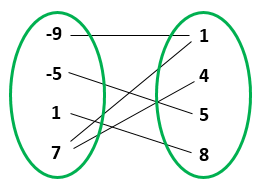Unlike the usual picture of a function:you probably don't want to imagine arrowheads on the line segments for a relation! You want to be able to just turn around your mental picture of a relation $$R \colon X \nrightarrow Y$$ and see the transpose $$R^\top \colon Y \nrightarrow X$$.

Of course each line segment represents a $$\texttt{true}$$ in the matrix I mentioned earlier, and each absence of a line segment represents a $$\texttt{false}$$. Composing relations has a very simple pictorial interpretation in terms of these line segments... and you can see that it's the same as matrix multiplication using $$\texttt{and}$$ and $$\texttt{or}$$.

I don't know if this helps, but I feel completely happy with my understanding of relations.... probably because I have a bunch of interlocking ways to think about them... so maybe I can make you happy too.Comment Source:Anindya wrote: > but tbh I don't really have much of a feel for what a relation is, beyond the formal definition. I tend to visualize relations as matrices of 0's and 1's, or matrices of \$$\texttt{true}\$$'s and \$$\texttt{false}\$$'s. Composing relations is just matrix multiplication using \$$\texttt{and}\$$ and \$$\texttt{or}\$$ instead of \$$\times\$$ and \$$+\$$. Profunctors are also just glorified matrices, with composition being done by a version of matrix multiplication. Another way I imagine a relation is this. A set is a bunch of dots. A relation from a set \$$X\$$ to a set \$$Y\$$ is as a bunch of line segments: one joining each dot in \$$X\$$ to each dot in \$$Y\$$ to which its related: <center><img src = "https://study.com/cimages/multimages/16/relation6.jpg"></center> Unlike the usual picture of a _function_: <center><img src = "https://upload.wikimedia.org/wikipedia/commons/thumb/8/83/Injection_keine_Injektion_2a.svg/300px-Injection_keine_Injektion_2a.svg.png"></center> you probably don't want to imagine _arrowheads_ on the line segments for a relation! You want to be able to just turn around your mental picture of a relation \$$R \colon X \nrightarrow Y\$$ and see the transpose \$$R^\top \colon Y \nrightarrow X\$$. Of course each line segment represents a \$$\texttt{true}\$$ in the matrix I mentioned earlier, and each _absence_ of a line segment represents a \$$\texttt{false}\$$. Composing relations has a very simple pictorial interpretation in terms of these line segments... and you can see that it's the same as matrix multiplication using \$$\texttt{and}\$$ and \$$\texttt{or}\$$. I don't know if this helps, but I feel completely happy with my understanding of relations.... probably because I have a bunch of interlocking ways to think about them... so maybe I can make you happy too. <img src = "http://math.ucr.edu/home/baez/emoticons/tongue2.gif"> 
• Options
18.
edited August 2018

Pierre wrote:

In your perception, tensoring x composing (and or series x parallel) could be framed as related to duality?

I said no in comment #10 when you asked that in comment #9, and I'll say it again: no.

You recently presented "Profunctor theory is to category theory as linear algebra is to set theory!". Is there some other analogies like this that you could present for us?

There are tons of analogies! My brain is packed with analogies. For example, in my previous two comments I explained the analogy between profunctors and relations, and the analogy between relations and matrices. Putting them together gives the analogy between profunctors and matrices, which is the reason profunctor theory is like linear algebra.

Of course, these analogies are only useful if you know exactly how they work and also how they don't work.

Every really good analogy is yearning to be a functor; when it's a functor we can work with it mathematically in a rigorous way.

Comment Source:Pierre wrote: > In your perception, tensoring x composing (and or series x parallel) could be framed as related to duality? I said no in comment #10 when you asked that in comment #9, and I'll say it again: no. > You recently presented "Profunctor theory is to category theory as linear algebra is to set theory!". Is there some other analogies like this that you could present for us? There are tons of analogies! My brain is packed with analogies. For example, in my previous two comments I explained the analogy between profunctors and relations, and the analogy between relations and matrices. Putting them together gives the analogy between profunctors and matrices, which is the reason profunctor theory is like linear algebra. Of course, these analogies are only useful if you know exactly how they work and also how they _don't_ work. Every really good analogy is yearning to be a functor; when it's a functor we can work with it mathematically in a rigorous way.
• Options
19.

Ok thanks a lot.

Comment Source:Ok thanks a lot.
• Options
20.

Fair.

Comment Source:Fair.
• Options
21.
edited August 2018

www.journals.plos.org/ploscompbiol/article?id=10.1371/journal.pcbi.1005683

just found it..on the frame of analogies..

Comment Source:www.journals.plos.org/ploscompbiol/article?id=10.1371/journal.pcbi.1005683 just found it..on the frame of analogies..
• Options
22.
edited September 2018

Speaking of analogies, I had an insight regarding the relation between profunctors and electrical circuits, that helped me to intuit Dan's answer to the puzzle. Each profunctor (or tensored pair of profunctors) can be modeled by a circuit, and circuits compose. $$\mathcal{V}$$-enrichment means we can "measure" a value in $$\mathcal{V}$$ for objects in a category (the hom-functor), and profunctors allow us to measure those values across (tensored) categories. As Anindya said, this is like creating a bridge between categories - just as we can measure current between any two nodes in a circuit (even if the two nodes belong to different circuits themselves).

Consider also the principle that the flow of current is inversely proportional to resistance (i.e. Ohm's law https://en.wikipedia.org/wiki/Ohm's_law]). This seems related to the quantale nature of $$\mathbf{Prof}_\mathcal{V}$$, namely the path of least resistance is a join. I'm certain this has been elaborated much further here: https://arxiv.org/abs/1504.05625

This leads to a formulation of the puzzle as: prove that the snake equation forms a circuit whose end-to-end value in $$\mathcal{V}$$ is the same as measuring the value across the identity profunctor. Something like the following:Comment Source:Speaking of analogies, I had an insight regarding the relation between profunctors and electrical circuits, that helped me to intuit Dan's answer to the puzzle. Each profunctor (or tensored pair of profunctors) can be modeled by a circuit, and circuits compose. \$$\mathcal{V}\$$-enrichment means we can "measure" a value in \$$\mathcal{V}\$$ for objects in a category (the hom-functor), and profunctors allow us to measure those values across (tensored) categories. As Anindya said, this is like creating a bridge between categories - just as we can measure current between any two nodes in a circuit (even if the two nodes belong to different circuits themselves). Consider also the principle that the flow of current is inversely proportional to resistance (i.e. Ohm's law https://en.wikipedia.org/wiki/Ohm%27s_law]). This seems related to the quantale nature of \$$\mathbf{Prof}_\mathcal{V}\$$, namely the path of least resistance is a join. I'm certain this has been elaborated much further here: https://arxiv.org/abs/1504.05625 This leads to a formulation of the puzzle as: prove that the snake equation forms a circuit whose end-to-end value in \$$\mathcal{V}\$$ is the same as measuring the value across the identity profunctor. Something like the following: <a href="https://softwarethoughts.com/images/snake-equation.png"><img src="https://softwarethoughts.com/images/snake-equation.png"></a>
• Options
23.

Interesting @DennisYao...thanks

Comment Source:Interesting @DennisYao...thanks
• Options
24.

Actually, Lecture 68 and 69 (with the associated comments) really helps clear it up more for me. It pays to read in order! Scott's comment was especially clarifying for the circuit metaphor (profunctors are machines that need an external energy source). Also Anindya's proof of the snake equations is a good companion to Dan's answer in this thread.

Comment Source:Actually, Lecture 68 and 69 (with the associated comments) really helps clear it up more for me. It pays to read in order! [Scott's comment](https://forum.azimuthproject.org/discussion/comment/20453/#Comment_20453) was especially clarifying for the circuit metaphor (profunctors are machines that need an external energy source). Also Anindya's [proof of the snake equations](https://forum.azimuthproject.org/discussion/comment/20462/#Comment_20462) is a good companion to Dan's answer in this thread.
• Options
25.

ok...

Comment Source:ok...